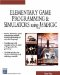# GetDistance

This command gives the distance between two objects. The syntax is

` odist = object.GetDistance(Object2); `

where odist is the distance between object and object2 .

### Notes

Another way to express this command would be to say,

` odist = object.GetDistance(x, y, z); `

where x , y , and z are points in 3D space, and odist is the distance from that point to object .

### Sample Code

` oworld = New World(); ocamera = New Camera(oworld); sphere = oworld.CreateSphere(50,50,50,25,25); //Create second sphere. Sphere2 = oworld.CreateSphere(10,10,10,10,10); ocamera.MoveBack(1200); oCamera.Walk(); sphere.AddPosition(100,200,30,5); //Move it in 5 seconds. // Other sphere follows it. Sphere2.Follow(sphere,50,60,10,10,10); //Loop While(1) {  SetText(sphere2.GetDistance(sphere)); } `Elementary Game Programming & Simulations Using Jamagic (Charles River Media Game Development)
ISBN: 1584502614
EAN: 2147483647
Year: 2002
Pages: 105
Authors: Sergio Perez